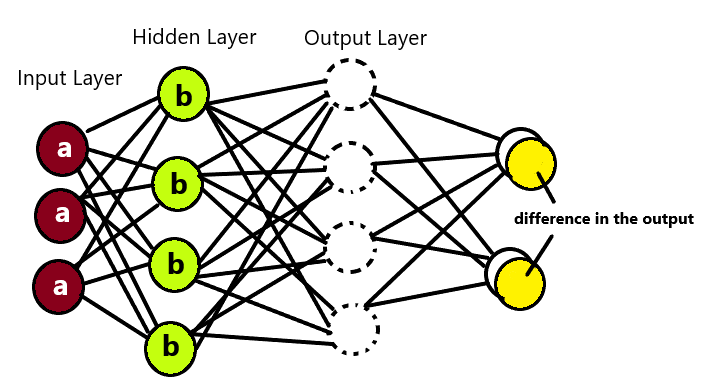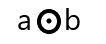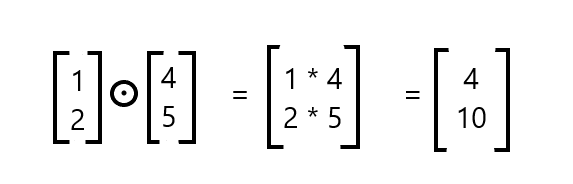×

Search anything:

# Back Propagation (Intuitively)

#### Machine Learning (ML)Get this book -> Problems on Array: For Interviews and Competitive Programming

Back Propagation is one of the most important topics of Neural Net Training. It is the process of tuning the weights of neural network and is based on the rate of error of previous epoch. If we tune the weights properly then we can ensure the low error rates.

Back propagation started to create a buzz after a paper by David Rumelhart, Geoffrey Hinton, and Ronald Williams was published in 1986. It is the short form of "back propagation of errors".This led to a revolution because the neural net problems could be easily solved using back propagation algorithm.
In this algorithm we only refer to the algorithm for computing gradient. We do not calculate the use of the gradient.

Feedforward neural network is the artificial neural network in which the nodes never form a cycle. It has an input layer, a hidden layer and an output layer.

As the efficiency increases, back propagation can compute gradient of loss function. It is majorly used for the feedforward neural networks. Back propagation is all about the partial derivative of the of the cost function with respect to any weight in the network. This told us about the change in the cost when we changed the weights or bias. This expression gave the intuitive approach to the back propagation.

Below diagram explains the working of the back propagation:Firstly, the input arrives via preconnected path. Then the input will be modeled via the weights b, selected randomly. Then we will calculate the output for each neuron. This will start from input layer, then go to the hidden layer and end at output layer. We can clearly calculate the differences in the output by subtracting actual and desired output.
Then we will go from output layer back to the hidden layers to correct the output by adjusting weights.

Backpropagation simplifies the network by using the weighted links. They have least effect on the trained networks. This is quite helpful for the neural networks that are error sensitive like speech recognition and image recognition. Backpropagation can function with any number of inputs. It uses the chain and power mechanism.

The main goal of this algorithm is to compare the partial derivatives of bias and weight with Cost function in a given network.
The back propagation is based on the linear algebra operations like vector multiplication, vector addition and much more. For example a and b are two vectors. Hence to represent their vector product we would use the below notation:The components are as follows:This product done element by element is known as hadamard product or the Schur Product. It comes handly when implementing the back propagation.

Backpropagation is fast and simple. It has no parameters to tune except the inputs. Since feedforward is the simplest and the first neural network, it is widely used.

Back propagations are of two main types:

• Static
• Recurrent

The static back propagation creates the mapping of the static input for the static output. However, the recurrent back propagation is fed forward till the value is reached. The static back propagation is faster than the recurrence back propagation.

• The actual performance sometimes depends on the input data.
• Avoid the mini batch approach. Rather prefer the matrix approach.
• It can be sensitive to the noisy data.#### Srishti Guleria

Srishti Guleria has been a Technical Author, Software Developer, Intern at OpenGenus and a Computer Science student at Rajasthan Technical University, Kota